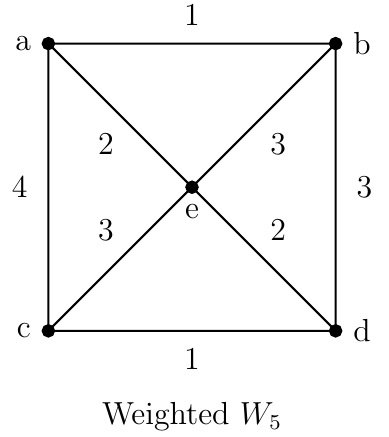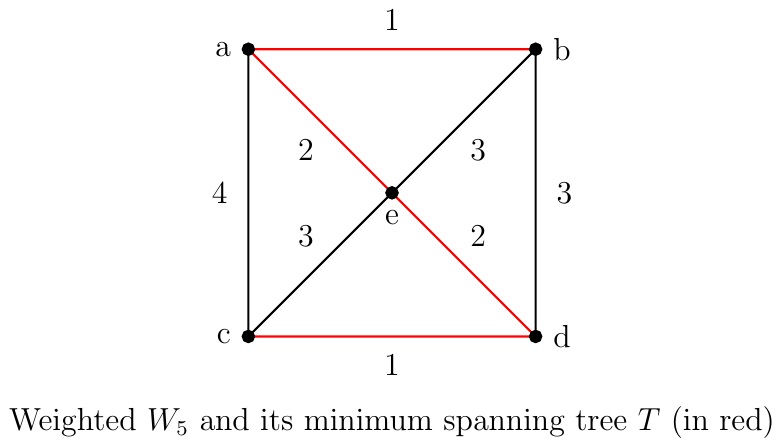Discrete Math Home

## Minimum Spanning Trees

Definition (Minimum spanning tree). A minimum spanning tree of a connected weighted graph $$G$$ (i.e., edges of $$G$$ have weights) is a spanning tree whose sum of edge weights is not greater than that of other spanning trees of $$G$$.

There are two well-known algorithms to find a minimum spanning tree: Prim's algorithm (1957) and Kruskal's algorithm (1956). An algorithm is greedy if it makes optimal choices at each step. A greedy algorithm does not necessarily produce an optimal solution. Both Prim's algorithm and Kruskal's algorithm are greedy algorithms but produce optimal solutions.

Prim's algorithm
Input: a weighted connected graph $$G$$ on $$n$$ vertices
Output: $$T$$, a minimum spanning tree of $$G$$

$$T=$$ a minimum weight edge of $$G$$
for $$i=1$$ to $$n-2$$
$$e=$$ an edge of minimum weight incident with a vertex in $$T$$
not forming a cycle if added to $$T$$
$$T=T+e$$
return $$T$$Example. Use Prim's algorithm to find a minimum spanning tree of the above weighted $$W_5$$.

Solution. We get a minimum spanning tree $$T$$ by the following steps.

• $$T=\{a,b\}$$ (or $$\{c,d\}$$).

• $$\{c,a\},\{e,a\},\{e,b\}, \{d,b\}$$ are all incident with one vertex of $$T$$, not forming a cycle when added to $$T$$, with weights $$4,2,3,3$$ respectively.

• $$T=T+\{e,a\}$$.

• $$\{c,a\},\{c,e\},\{d,e\}, \{d,b\}$$ are all incident with one vertex of $$T$$, not forming a cycle when added to $$T$$, with weights $$4,3,2,3$$ respectively.

• $$T=T+\{d,e\}$$.

• $$\{c,a\},\{c,e\},\{c,d\}$$ are all incident with one vertex of $$T$$, not forming a cycle when added to $$T$$, with weights $$4,3,1$$ respectively.

• $$T=T+\{c,d\}$$.

Thus a minimum spanning tree $$T$$ is the walk $$baedc$$ with weight 6.Kruskal's algorithm is similar to Prim's algorithm. But it does not require the new edge at each step to be incident with a vertex of the preceding tree which means we may get a forest at an interim step.

Kruskal's algorithm
Input: a weighted connected graph $$G$$ on $$n$$ vertices
Output: $$T$$, a minimum spanning tree of $$G$$

$$T=$$ the empty graph
for $$i=1$$ to $$n-1$$
$$e=$$ an edge of minimum weight not forming a cycle if added to $$T$$
$$T=T+e$$
return $$T$$

Example. Use Kruskal's algorithm to find a minimum spanning tree of the above weighted $$W_5$$.

Solution. We get a minimum spanning tree $$T$$ by the following steps.

• $$T=$$ the empty graph.

• $$\{a,b\}$$ and $$\{c,d\}$$ are the edges of minimum weight.

• $$T=T+\{c,d\}$$ (or $$T+\{a,b\}$$).

• $$\{a,b\}$$ is the edge of minimum weight that does not form a cycle when added to $$T$$.

• $$T=T+\{a,b\}$$.

• $$\{e,d\}$$ (or $$\{a,e\}$$) is the edge of minimum weight that does not form a cycle when added to $$T$$.

• $$T=T+\{e,d\}$$.

• $$\{a,e\}$$ is the edge of minimum weight that does not form a cycle when added to $$T$$.

• $$T=T+\{a,e\}$$.

Thus a minimum spanning tree $$T$$ is the walk $$baedc$$ with weight 6.

Last edited